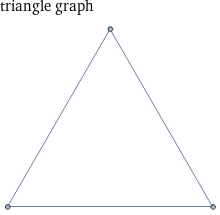Search IntMath
Close

450+ Math Lessons written by Math Professors and Teachers

5 Million+ Students Helped Each Year

1200+ Articles Written by Math Educators and Enthusiasts

Simplifying and Teaching Math for Over 23 Years

# Equiangular Triangles in Geometry## What are Equiangular Triangles?

In geometry, an equiangular triangle is a triangle that has three angles of equal measure. All three angles of an equiangular triangle measure 60 degrees, making it an equilateral triangle as well. This means that all three sides of the triangle are equal in length, and all three angles are equal in measure. This type of triangle is also known as an equilateral triangle.

An equiangular triangle can also be referred to as an "equilateral triangle" or "equilateral polygon". This means that all sides of the triangle are equal in length, and all three angles are equal in measure. The three angles of an equiangular triangle always measure 60 degrees.

## Properties of Equiangular Triangles

The most obvious property of an equiangular triangle is that all three angles are equal. This makes the triangle unique from other types of triangles, such as isosceles or scalene triangles. All three angles measure 60 degrees, meaning all three sides of the triangle are equal in length.

Since all three angles are equal, the triangle is also equilateral. This means that all three sides of the triangle are of equal length. An equiangular triangle is also a regular polygon, meaning all sides and angles are of equal length. This makes the triangle a perfect shape for use in mathematics and geometry.

## Examples of Equiangular Triangles

Equiangular triangles can be found in many different places in mathematics and geometry. The most common example is the equilateral triangle, which can be used to construct regular polygons. This type of triangle is also used to construct regular hexagons, octagons, and other shapes.

In addition to the equilateral triangle, equiangular triangles are also used in the construction of rhombuses and kites. These shapes are also regular polygons, and are made up of four equal sides and angles. The angles of a rhombus or kite always measure 90 degrees, making it an equiangular triangle as well.

## Practice Problems

Now that you know the basics of equiangular triangles, let's take a look at some practice problems. See if you can figure out the answers to these questions!

1. What is the measure of the angles of an equiangular triangle? Answer: All three angles of an equiangular triangle measure 60 degrees.

2. What type of triangle is an equiangular triangle? Answer: An equiangular triangle is also known as an equilateral triangle or equilateral polygon.

3. What type of polygons can be constructed with equiangular triangles? Answer: Equiangular triangles can be used to construct regular polygons, such as equilateral triangles, rhombuses, and kites.

4. Are all polygons created with equiangular triangles regular? Answer: Yes, all polygons created with equiangular triangles are regular, meaning all sides and angles are equal in measure.

5. Are equiangular triangles the same as isosceles triangles? Answer: No, equiangular triangles are not the same as isosceles triangles. Equiangular triangles have all three angles measuring 60 degrees, while isosceles triangles have two angles measuring the same and one angle measuring a different measure.

6. How many sides does an equiangular triangle have? Answer: An equiangular triangle has three sides.

## Summary

In this article, we discussed equiangular triangles in geometry. We explored what an equiangular triangle is, its properties, and some examples of where it is used. We also looked at some practice problems to test your knowledge of equiangular triangles. Remember, an equiangular triangle has three angles measuring 60 degrees, making it an equilateral triangle as well.

## FAQ

### What is an equiangular triangle?

An equiangular triangle is a triangle with all three angles measuring the same. In other words, every angle of an equiangular triangle is equal to 60 degrees.

### What is the sum of the angles in an equiangular triangle?

The sum of the angles in an equiangular triangle is 180 degrees.

### What is the area of an equiangular triangle?

The area of an equiangular triangle is equal to (s^2 v3)/4, where s represents the length of each side.

## Problem SolverThis tool combines the power of mathematical computation engine that excels at solving mathematical formulas with the power of GPT large language models to parse and generate natural language. This creates math problem solver thats more accurate than ChatGPT, more flexible than a calculator, and faster answers than a human tutor. Learn More.